# Double Pendulum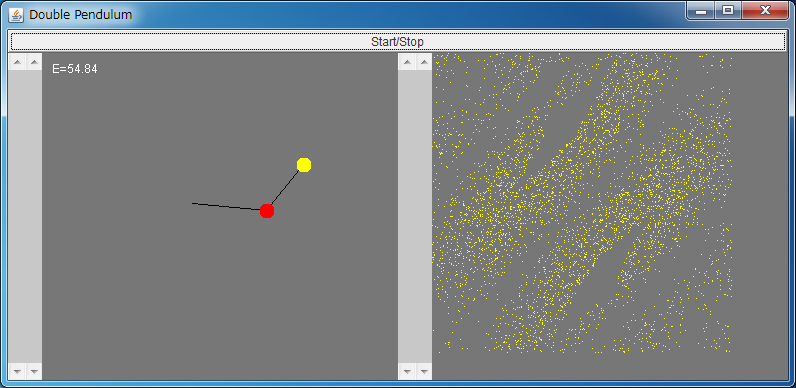If the above application does not start, please install OpenJDK from adoptium.net.

[Sample images of double pendulum simulator]
• E=101.52
• Torus ( 1 / 2 )
• Chaos ( 1 )
• E=11.4
• Torus ( 1 / 2 / 3 )
• Chaos ( 1 )

Let us consider a double pendulum as shown in a below figure.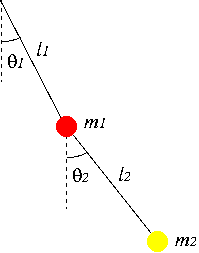Although the double pendulum is often introduced in many textbooks of the classical mechanics, its dynamics are seldom analyzed in them. Actually, it is known that it easily exhibits chaotic behaviors. It is important that chaos appears even in a simple model that is treated in the textbooks.

In the double pendulum, the effect of the friction around the axis of rotation is not considered. Therefore, the energy of the system is conserved, and such a system is called a Hamiltonian system or a conservative system.

In dissipative systems, where the energy of the system is not conserved, e.g., in Hénon map and Lorenz system, there exists a chaotic attractor for the appropriate values of the parameters. However, in the Hamiltonian system such as the double pendulum, there are not chaotic attractors, but there exist the invariant tori, the chaotic sea, and so on, depending on the energy and the initial conditions. For further information on the dynamics in the Hamiltonian system, please see the standard map simulator.

The energy E of the system is a sum of the kinetic energy K and the potential energy U written as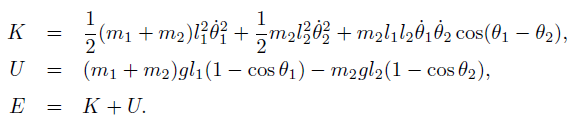Using the Lagrange differential equation, a set of differential equations which governs the dynamics of the double pendulum is obtained, and it is written as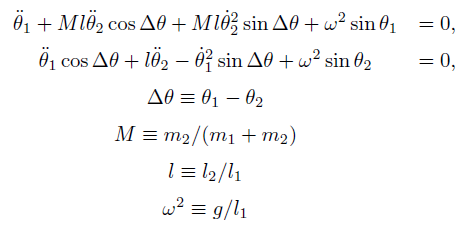From the above equations, the second derivatives of angles are obtained as follows.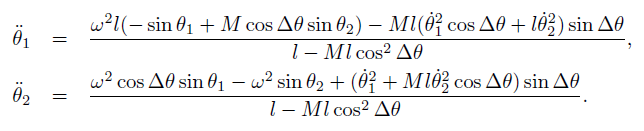Regarding the above differential equations as a differential equation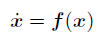for a vector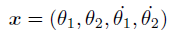, behaviors of a double pendulum can be analyzed.

Because the double pendulum is a Hamiltonian system (a conservative system) where the energy of the system is conserved, one must use numerical integration methods which conserve the energy.

Here we used the fourth order implicit Gaussian method written as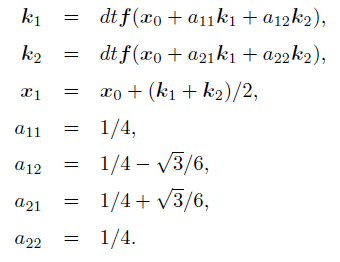On the left side, the behaviors of the double pendulum is displayed. After stopping the dynamics with the top button, angles and velocities can be configured.
On the right side, a Poincaré section of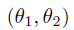where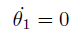is displayed.